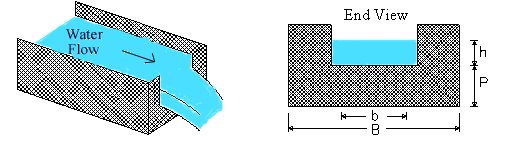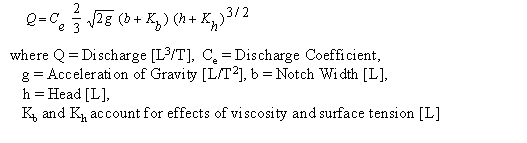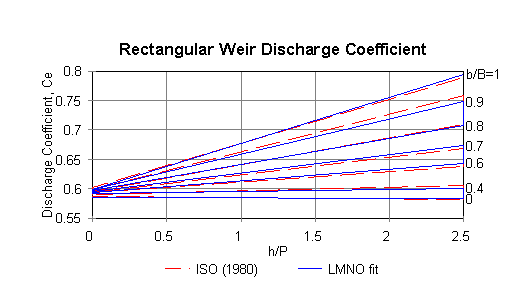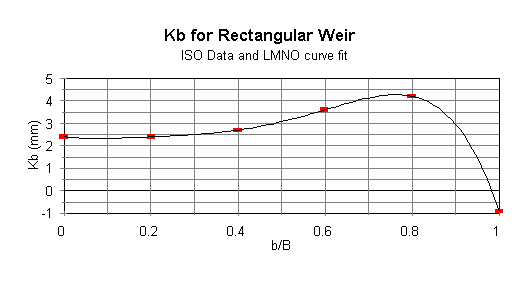Rectangular Weir Calculator

Discharge, Head, and Design Calculations. Equations and Installation Guidelines

Register to enable "Calculate" button.

 Solve for: Discharge Head Notch Width Discharge, Q: ft3/s (cfs) gal/min (gpm) m3/s Notch Width, b: inch ft cm m Channel Width, B: inch ft cm m Head, h: inch ft cm m Crest Height, P: inch ft cm m b/B ratio: h/P ratio: Discharge Coefficient, Ce: Kb: m © 2014 LMNO Engineering, Research, and Software, Ltd. http://www.LMNOeng.com
Units in rectangular weir calculation: cm=centimeter, ft=foot, gal=US gallon, m=meter, min=minute, s=second.Rectangular weir topics:  Introduction    Equations     Installation Guidelines and Equation Applicability     Error Messages     References

Introduction
The rectangular weir is the most commonly used thin plate weir.  Weirs are typically installed in open channels such as streams to determine discharge (flowrate).  The basic principle is that discharge is directly related to the water depth (h) in the figure above; h is known as the "head."  Rectangular weirs can be "suppressed," "partially contracted," or "fully contracted."   Suppressed means there are no contractions.  A suppressed weir's notch width (b) is equal to the channel width (B); thus, there really isn't a notch - the weir is flat all the way along the top.  For a weir to be fully contracted, (B-b) must be greater than 4hmax, where hmax is the maximum expected head on the weir (USBR, 1997).  A partially contracted weir has B-b between 0 and 4hmax.  Weir contractions cause the water flow lines to converge through the notch.

USBR (1997) provides equations for a "standard" fully contracted rectangular weir and a "standard" suppressed weir.  The U.S. Bureau of Reclamation has conducted many weir tests over several decades using weirs with particular dimensions - usually b's in 1 ft. increments up to about 10 ft.  Therefore, any weir outside their tested dimensions is non-standard, and their equations should not be used.  To provide a single reliable, accurate method to model all rectangular weirs (suppressed, partially contracted, and fully contracted), the Kindsvater-Carter equation (Kindsvater and Carter, 1959) was developed.  It is considerably more complex than the USBR standard weir equations.  However, USBR (1997) states that the Kindsvater-Carter method is at least as accurate, if not more, than the standard weir equations for suppressed and fully contracted weirs.  And further, the Kindsvater-Carter equation reliably models partially contracted weirs.  ISO (1980), ASTM (1993), and USBR (1993) all recommend using the Kindsvater-Carter method for all rectangular weirs.

Rectangular Weir Equation
The Kindsvater-Carter rectangular weir equation (ISO, 1980):The sum b+Kb is called "effective width" and the sum h+Kh is called "effective head."  The value for g is 9.8066 m/s2 and Kh=0.001 m.  Ce is a function of b/B and h/P, and Kb is a function of b/B.  Our "Solve for Flowrate" calculation is analytic, but our "Solve for Head" and "Solve for Notch Width" calculations require numerical solutions since Ce and Kb cannot be computed directly, as they are functions of h and/or b.

ISO (1980) provides a graph and equations for Ce vs. h/P for b/B=0, 0.2, 0.4, 0.5, 0.6, 0.7, 0.8, 0.9, and 1.0.  To account for other b/B values, LMNO Engineering developed an equation for all b/B values.  The LMNO Engineering curves are plotted below with the ISO curves, so you can see our goodness of fit.   Likewise, ISO (1980) provides values of Kb for b/B=0, 0.2, 0.4, 0.6, 0.8, and 1.0, and LMNO Engineering fit an equation through the data to facilitate solving numerically for b.  The LMNO Engineering fit is shown below with the ISO data.  Our numerical solutions utilize a cubic solver routine.  The computations are performed in double precision (the calculations on all of our web pages use double precision).Rectangular Weir Installation Guidelines and Equation Applicability             Top of Page
(from ISO (1980) unless otherwise indicated)
Head (h) should be measured upstream from the weir at a distance of 4-5 times the maximum head.

It doesn't matter how thick the weir is except where water flows through the notch.  The weir should be between 1 and 2 mm thick in the opening.  If the bulk of the weir is thicker than 2 mm, the downstream edge of the opening can be chamfered at an angle greater than 45o to achieve the desired thickness of the edges.  You want to avoid having water cling to the downstream face of the weir.

Water surface downstream of the weir should be at least 0.2 ft. (6 cm) below the weir crest (i.e. below the bottom of the opening) (USBR, 1993).

Measured head (h) should be ≥ 0.03 m (1.2 inch).

P is measured from the bottom of the upstream channel and should be ≥ 0.1 m (3.9 inch).

The notch width (b) and channel width (B) should be ≥ 0.15 m (5.9 inch).

0 < b/B ≤ 1  and  0 < h/P ≤ 2.5.

If b<B (i.e. contracted weir), then  (B-b) ≥ 0.2 m (3.9 inch).

Error Messages given by calculation                                Top of Page
"All inputs must be positive".  This is an initial check of user input.

"h must be ≥0.03 m", "P must be 0.1 to 10000 m", "h/P must be ≤2.5", "b must be ≥ 0.15 m", "B must be 0.15 to 10000 m", or "b/B must be ≤1".  These are initial checks of user input.  Though ISO (1980) does not place an upper limit on P or B, our calculation imposes limits of 10000 m for practicality.  For actual weirs, P and B generally don't exceed several meters.

"h will be <0.03 m", "h will be >2.5P", "b will be <0.15 m", or "b will be >B".  Inputs are such that the computed parameter will be out of range.

"Infeasible input".  Inputs are such that the computed parameter will be out of range.

References
ASTM (1993).  American Society for Testing and Materials.  ASTM D5242.   Standard method for open-channel flow measurement of water with thin-plate weirs.   1993.  Available from Global Engineering Documents at http://global.ihs.com

ISO (1980).  International Organization of Standards.  ISO 1438/1-1980(E).   Water flow measurement in open channels using weirs and venturi flumes - Part 1: Thin plate weirs.  1980.  Available from Global Engineering Documents at http://global.ihs.com

Kindsvater, C. E. and R. W. Carter.  1959.  Discharge characteristics of rectangular thin-plate weirs.  Transactions, American Society of Civil Engineers.   v. 24.  Paper No. 3001.

USBR (1997).  U.S. Department of the Interior, Bureau of Reclamation.  Water Measurement Manual.  1997.  3ed.  Available from http://www.usbr.gov/tsc/techreferences/mands/wmm/index.htm .

LMNO Engineering, Research, and Software, Ltd.
7860 Angel Ridge Rd.   Athens, Ohio 45701  USA   Phone: (740) 707-2614
LMNO@LMNOeng.com    http://www.LMNOeng.com

To:

Related calculations:

Weirs:
V-Notch
Cipoletti

End Depth Method: Circular
Rectangular
Triangular

Unit Conversions

Register